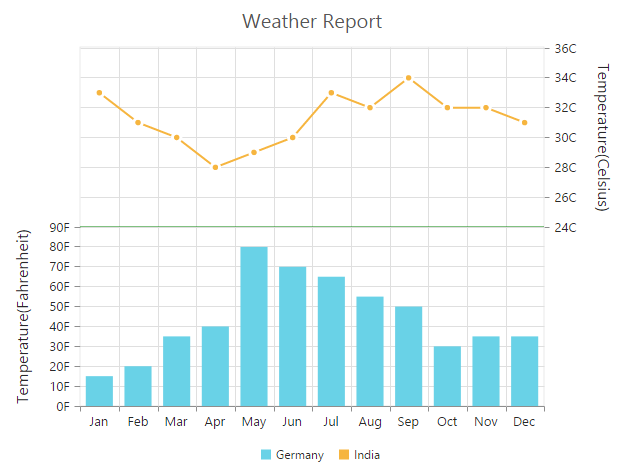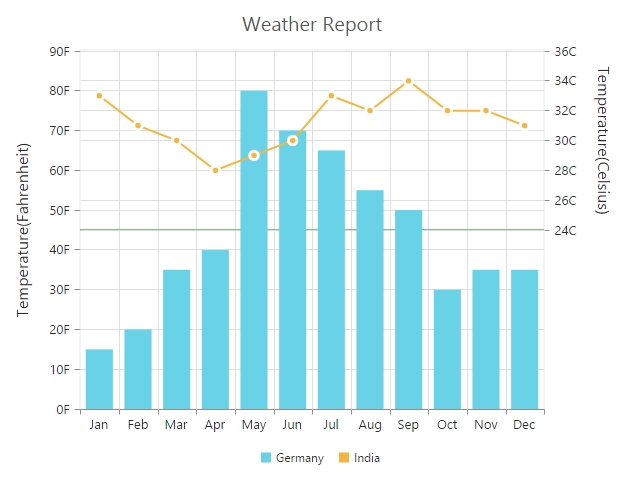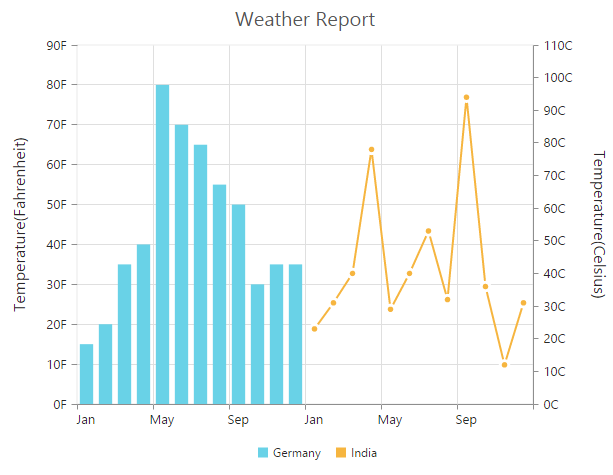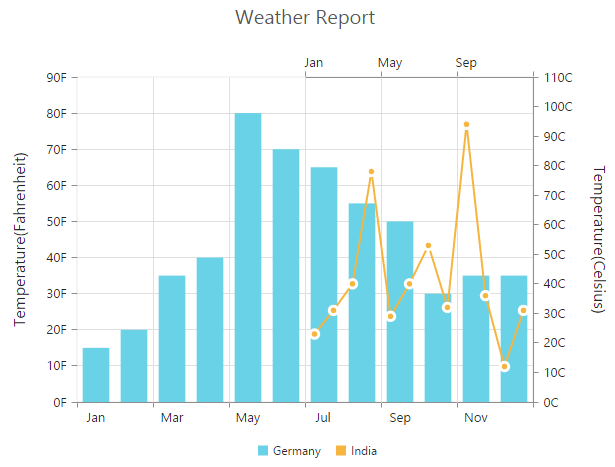# Multiple panes in JQuery Chart widget

Chart area can be divided into multiple panes using the `rowDefinitions` and `columnDefinitions` properties.

### Row Definitions

To split the chart area vertically into a number of rows, use `rowDefinitions` of the chart.

• javascript
• ``````\$("#container").ejChart({

//  Splitting chart area into multiple rows
rowDefinitions: [{
//  Split first row of the chart area
unit : 'percentage',
lineColor : 'Gray',
rowHeight : 50,
lineWidth : 0
}, {
//  Split second row of the chart area
unit : 'percentage',
lineColor : 'green',
rowHeight : 50,
lineWidth : 0
}],

axes: [{
//Create secondary axis and bind it to second row of chart area
name: "yAxis1",
rowIndex: 1
}],
series: [{

//Binding vertical axis name
yAxisName: "yAxis1",
// ...
}],
//  ...

});``````Row Span

For spanning the vertical axis along multiple panes vertically, you can use `rowSpan` property of axis.

• javascript
• ``````\$("#container").ejChart({

rowDefinitions: [{

// ...
},{

// ...
}],
axes: [{

// ...
}],

primaryYAxis: {
//  Span the PrimaryYAxis
rowSpan : 2,
},

series: [{
// ...
}],
//  ...

});``````## Column Definitions

To split the chart area horizontally into a number of columns, use `columnDefinitions` of the chart.

• javascript
• ``````\$("#container").ejChart({

//  Splitting chart area into multiple columns
columnDefinitions: [{
//  Split first column of the chart area
unit : 'percentage',
columnWidth : 50,
}, {
//  Split second column of the chart area
unit : 'percentage',
columnWidth : 50,
}],

axes: [{
//Create secondary axis and bind it to second column of chart area
name: "xAxis1",
columnIndex: 1
}],
series: [{

//Binding horizontal axis name
xAxisName: "xAxis1",
// ...
}],
//  ...

});``````Column Span

For spanning the horizontal axis along multiple panes horizontally, you can use `columnSpan` property of axis.

• javascript
• ``````\$("#container").ejChart({

columnDefinitions: [{

// ...
},{

// ...
}],
axes: [{

// ...
}],

primaryXAxis: {
//  Span the PrimaryXAxis
columnSpan : 2,
},

series: [{
// ...
}],
//  ...

});``````